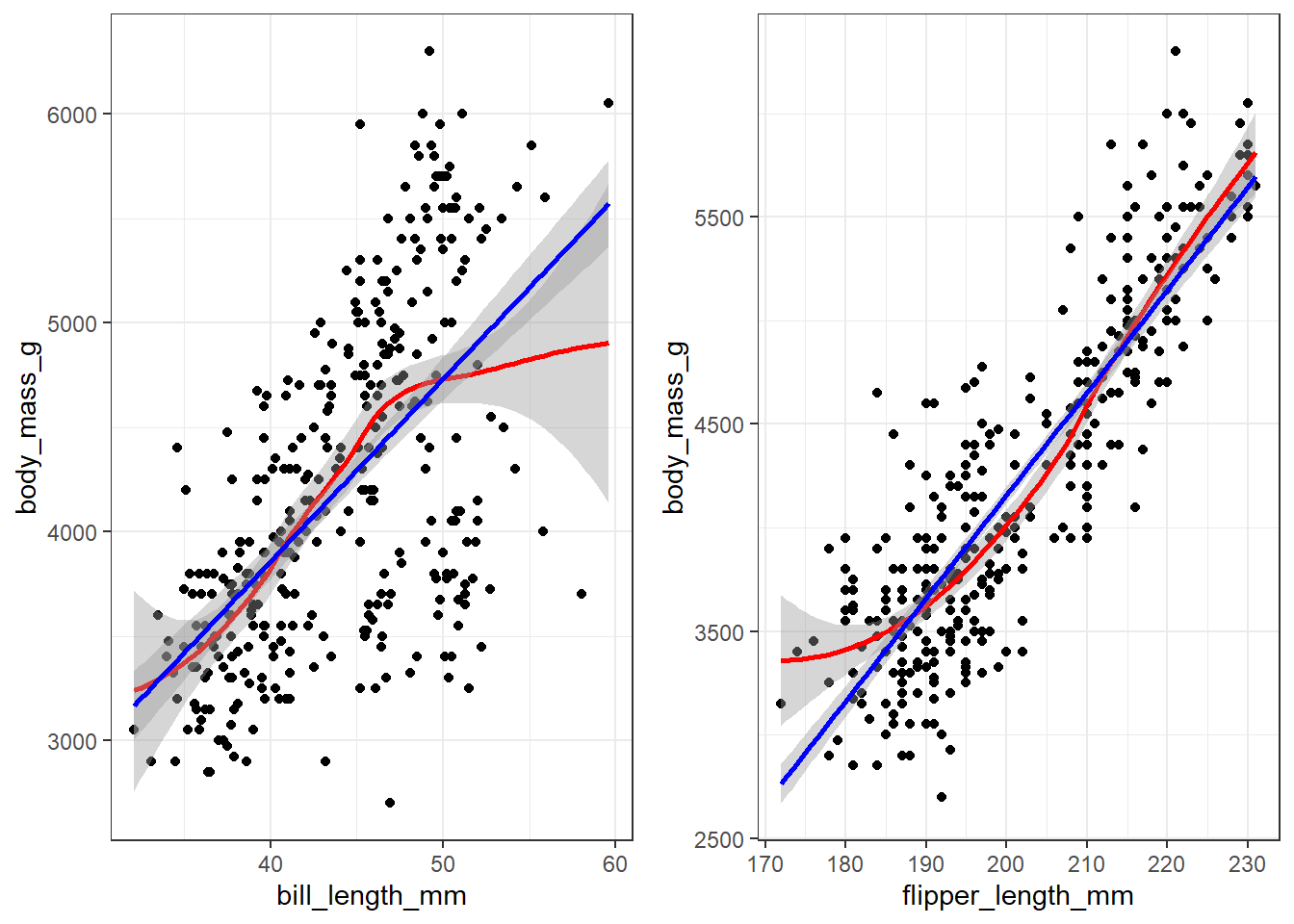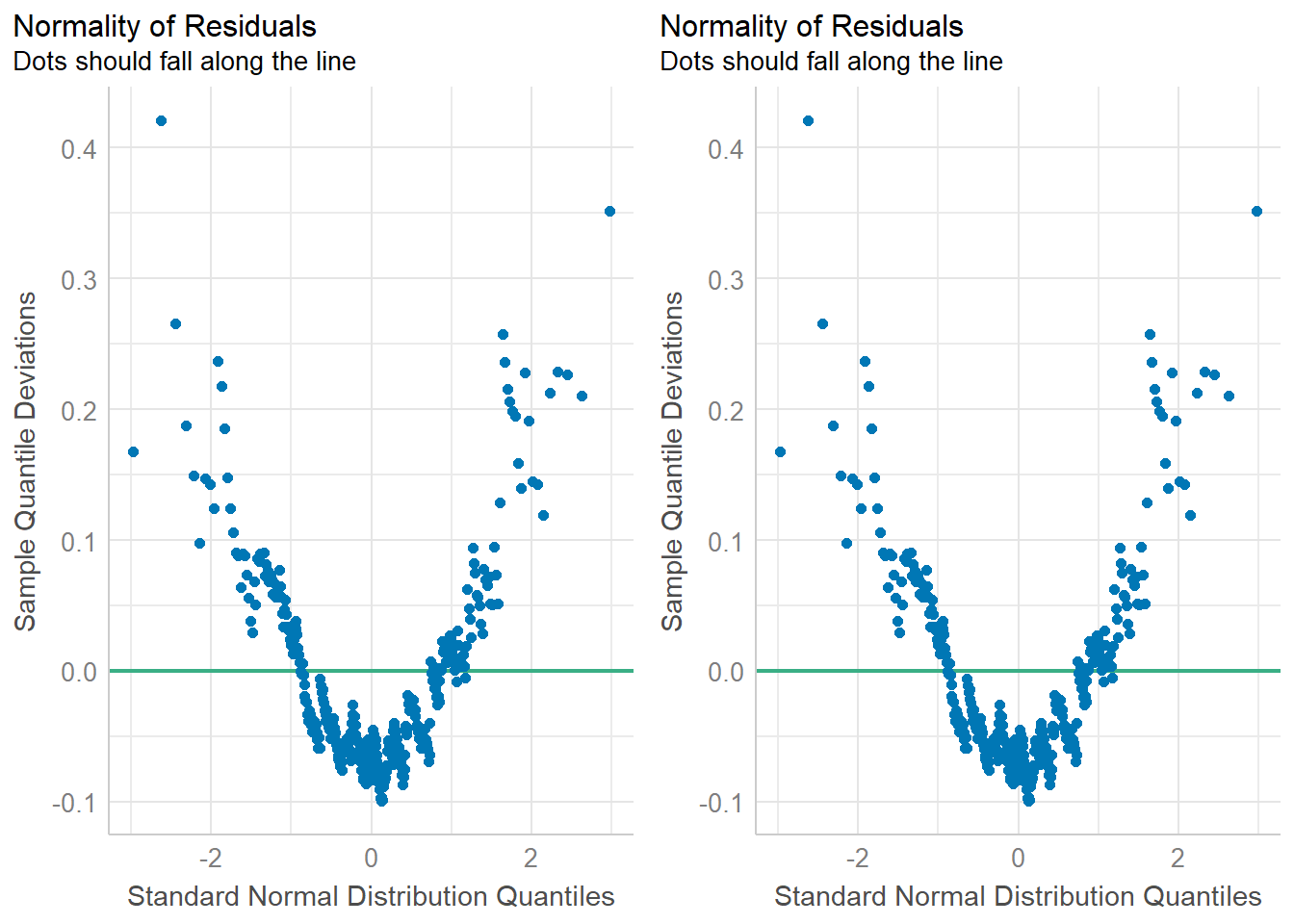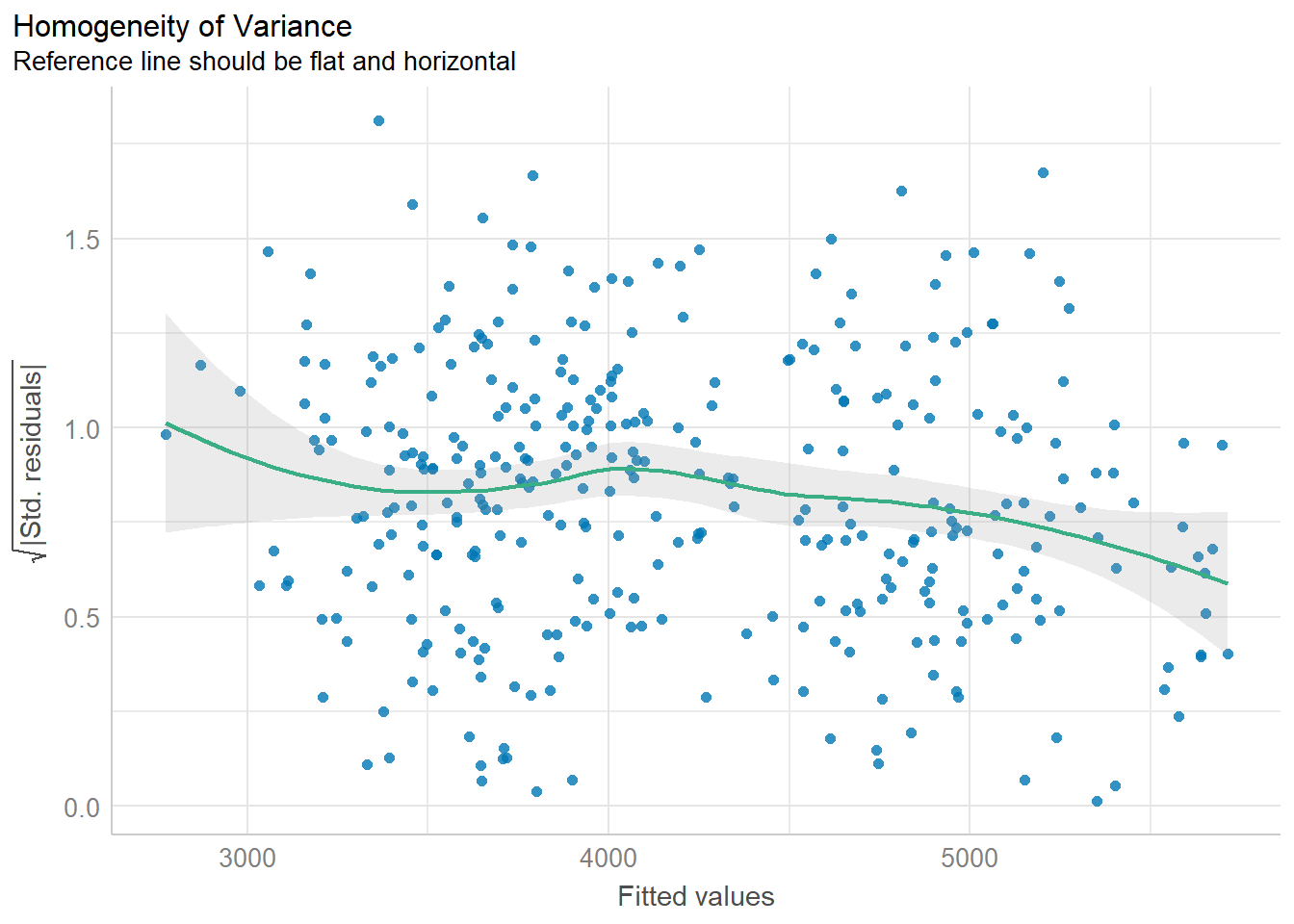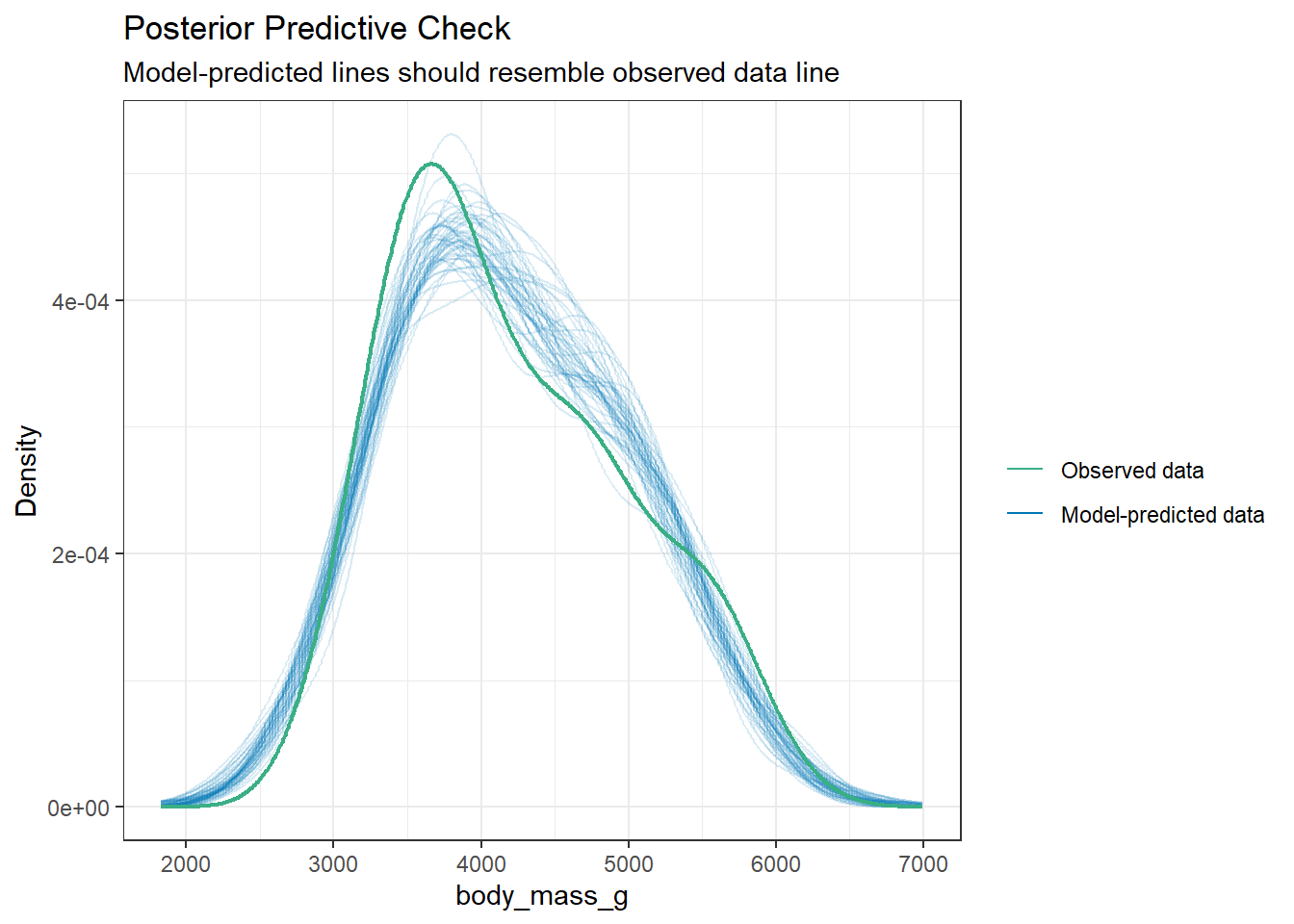## 10.6 Model Diagnostics

Recall from Section 7.2 the assumptions for linear regression model are;

• Linearity The relationship between $$x_j$$ and $$y$$ is linear, for all $$j$$.
• Normality, Homogeneity of variance The residuals are identically distributed $$\epsilon_{i} \sim N(0, \sigma^{2})$$
• Uncorrelated/Independent Distinct error terms are uncorrelated: $$\text{Cov}(\epsilon_{i},\epsilon_{j})=0,\forall i\neq j.$$

There are a few ways to visually assess these assumptions. We’ll look at this using a penguin model of body mass as an example.

pen.bmg.model <- lm(body_mass_g ~ bill_length_mm + flipper_length_mm, data=pen)

### 10.6.1 Linearity

Create a scatterplot with lowess AND linear regression line. See how close the lowess trend line is to the best fit line. Do this for all variables.

bill.plot  <- ggplot(pen, aes(y=body_mass_g, x=bill_length_mm)) +
geom_point() +   theme_bw() +
geom_smooth(col = "red") +
geom_smooth(method = "lm" , col = "blue")

flipper.plot  <- ggplot(pen, aes(y=body_mass_g, x=flipper_length_mm)) +
geom_point() +   theme_bw() +
geom_smooth(col = "red") +
geom_smooth(method = "lm" , col = "blue")

gridExtra::grid.arrange(bill.plot, flipper.plot, ncol=2)Both variables appear to have a mostly linear relationship with body mass. For penguins with bill length over 50mm the slope may decrease, but the data is sparse in the tails.

### 10.6.2 Normality of residuals.

There are two common ways to assess normality.

1. A histogram or density plot with a normal distribution curve overlaid.
2. A qqplot. This is also known as a ‘normal probability plot’. It is used to compare the theoretical quantiles of the data if it were to come from a normal distribution to the observed quantiles. PMA6 Figure 5.4 has more examples and an explanation.
gridExtra::grid.arrange(
plot(check_normality(pen.bmg.model)),
plot(check_normality(pen.bmg.model), type = "qq"),
ncol=2
)In both cases you want to assess how close the dots/distribution is to the reference curve/line.

### 10.6.3 Homogeneity of variance

The variability of the residuals should be constant, and independent of the value of the fitted value $$\hat{y}$$.

plot(check_heteroskedasticity(pen.bmg.model))This assumption is often the hardest to be fully upheld. Here we see a slightly downward trend. However, this is not a massive violation of assumptions.

### 10.6.4 Posterior Predictions

Not really an assumption, but we can also assess the fit of a model by how well it does to predict the outcome. Using a Bayesian sampling method, the distribution of the predictions from the model should resemble the observed distribution.

plot(check_posterior_predictions(pen.bmg.model))This looks like a good fit.

### 10.6.5 All at once

check_model(pen.bmg.model)Refer to PMA6 8.8 to learn about leverage.